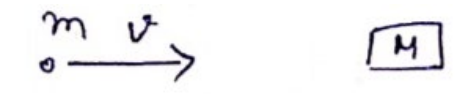# Solve the following :

Question:

A bullet of mass $m$ moving at a speed $v$ hits a ball of mass $M$ kept at rest. A small part having mass $m$ breaks from the ball and sticks to the bullet. The remaining ball is found to move at a speed of $v_{1}$ in the direction of bullet. Find the velocity of the bullet after the collision.

Solution:Use C.O.L.M,

$m v=\left(m^{\prime}+m\right) V_{2}+\left(M+m^{\prime}\right) V_{1}$

$V_{2}=\frac{m v-\left(M-m^{\prime}\right) V_{1}}{\left(m^{\prime}+m\right)}$